# Electric Field Lines by A.K.Sinha

Electric field lines & their properties :

Electric Field Lines :

Electric field lines or electric lines of force are imaginary lines which are used to show the direction of electric field intensity at a particular point around a source charge.

we may define an electric field line as a path, straight or curved such that tangent to it at any point gives the direction of electric field intensity at that point.

( Concept :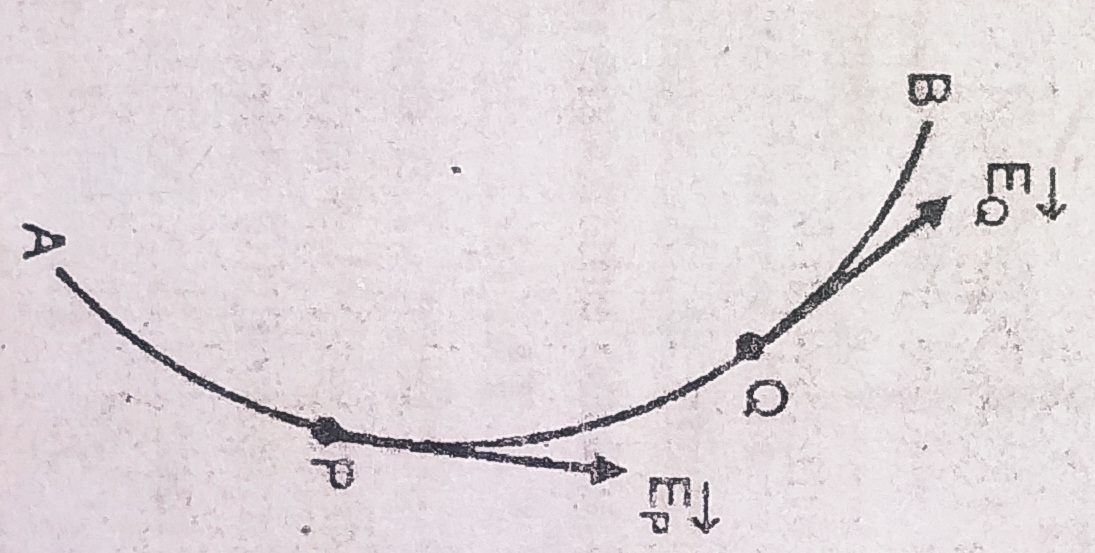FIG. 1

In above figure AB is an electric line of force. The tangent to the line at any point P gives us the direction of electric intensity EP at P. Similarly, tangent to AB at Q gives us the direction of EQ .

Properties of electric field lines :

• Perpendicular to the surface of conductor. Electric field lines are always perpendicular to the surface of any charge conductor.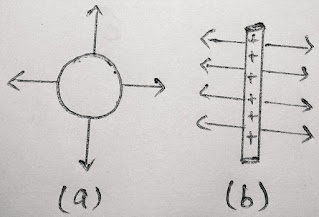• Intersect Never to each other. Because at the point of intersection there are two direction of electric intensity at the same point( as shown in fig. ) which is not possible.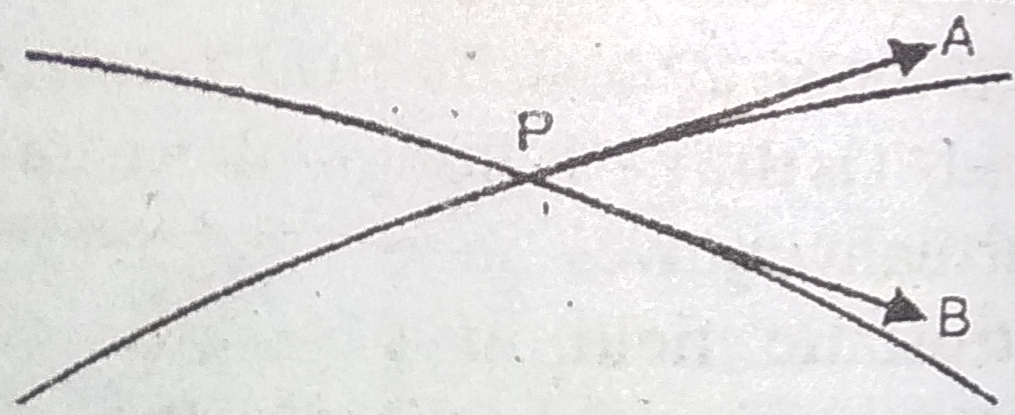• Tangent to the curve at any point gives the direction of electric field intensity at that point ( see point P & Q in FIG. 1 ).

• Intensity of field is maximum when charges are closely packed and minimum when loosely packed.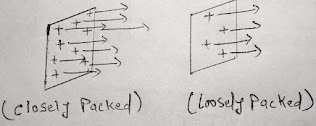• Contract longitudinally : The electric field lines contract longitudinally to show attraction between unlike charges.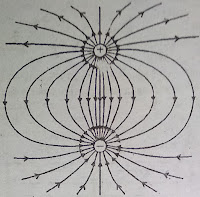• Exert lateral pressure : The electric field lines exert lateral pressure which show repulsion between like charges.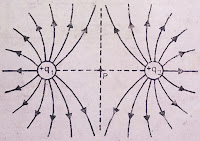( NOTE :  You can remember all the 7 properties by using words SPIT ICE as a trick. See the first bold letter of all 7 properties )

1.